Electron. J. Diff. Eqns., Vol. 2008(2008), No. 97, pp. 1-7.

### Positivity of the Green functions for higher order ordinary differential equations Michael I. Gil'

Abstract:
We consider the equation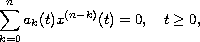where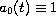,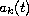(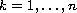) are real bounded functions. Assuming that all the roots of the polynomial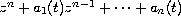(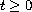) are real, we derive positivity conditions for the Green function for the Cauchy problem. We also establish a lower estimate for the Green function and a comparison theorem for solutions.

Submitted May 8, 2008. Published July 25, 2008.
Math Subject Classifications: 34C10, 34A40.
Key Words: Linear ODE; Green function; fundamental solution; positivity; comparison of solutions.

Show me the PDF file (203 KB), TEX file, and other files for this article.Michael I. Gil' Department of Mathematics Ben Gurion University of the Negev P.0. Box 653, Beer-Sheva 84105, Israel Email: gilmi@cs.bgu.ac.il